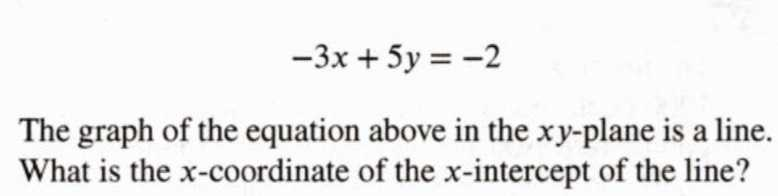### ¿Todavía tienes preguntas de matemáticas?

Pregunte a nuestros tutores expertos
Algebra
Pregunta$$- 3 x + 5 y = - 2$$

The graph of the equation above in the $$x y$$ -plane is a line. What is the $$x$$ -coordinate of the $$x$$ -intercept of the line?